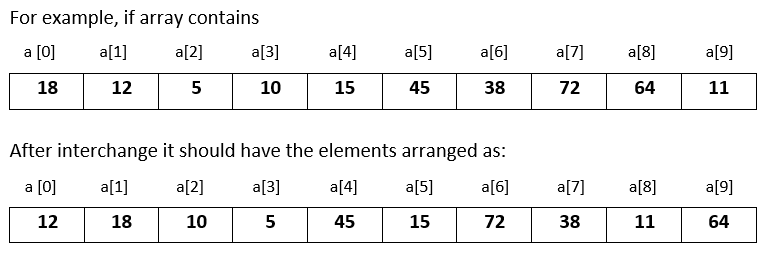# Input 10 numbers in integer array and interchange the consecutive numbers in Python

###### Arrays - Question 8

In this question, we will see how to input 10 numbers in integer array and interchange the consecutive numbers in it in Python programming. To know more about arrays click on the arrays lesson.

Q8) Write a program in Python to input 10 numbers in integer array and interchange the consecutive numbers in it. That is, interchange a with a, a with a, a with a and so on.#### Program

``````from array import *

t=0
a=array('i',[])

print('Enter 10 numbers')
for i in range(10):
a.append(int(input()))

for i in range(0,10,2):
t=a[i]
a[i]=a[i+1]
a[i+1]=t

print('\nModified array after interchanging the consecutive numbers');
for n in a:
print(n,end=' ')``````

#### Output

```Enter 10 numbers
18
12
5
10
15
45
38
72
64
11

Modified array after interchanging the consecutive numbers
12 18 10 5 45 15 72 38 11 64```